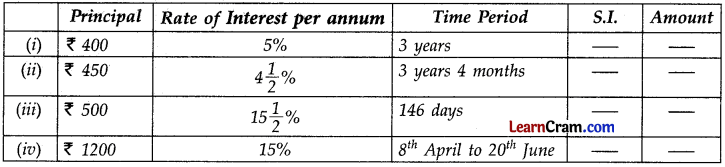# DAV Class 7 Maths Chapter 5 Worksheet 4 Solutions

The DAV Class 7 Maths Solutions and DAV Class 7 Maths Chapter 5 Worksheet 4 Solutions of Application of Percentage offer comprehensive answers to textbook questions.

## DAV Class 7 Maths Ch 5 WS 4 Solutions

Question 1.
Find the unknown quantity in each of the following.(i) P = ₹ 400, R = 5%, T = 3 years, S.I. = ?
S.I. = $$\frac{\mathrm{P} \times \mathrm{R} \times \mathrm{T}}{100}=\frac{400 \times 5 \times 3}{100}$$ = 60
Amount = P + S.I. = ₹ 400 + ₹ 60
= ₹ 460

P = ₹ 450, R = 4$$\frac{1}{2}$$%, = $$\frac{9}{2}$$%, T = 3 years 4 months, S.I. = ?
= 3 + $$\frac{4}{12}$$ = 3 + $$\frac{1}{3}=\frac{10}{3}$$ years
S.I = $$\frac{\mathrm{P} \times \mathrm{R} \times \mathrm{T}}{100}=\frac{450 \times 9 \times 10}{2 \times 3 \times 100}=\frac{135}{2}$$ = 67.50

(iii) P = ₹ 500, R = 15$$\frac{1}{2},=\frac{31}{2}$$%, = T = 146 days = $$\frac{146}{365}=\frac{2}{5}$$ years, S.I. = ?
S.I = $$\frac{\mathrm{P} \times \mathrm{R} \times \mathrm{T}}{100}=\frac{500 \times 31 \times 2}{2 \times 5 \times 100}$$ = ₹ 31
Amount = P + S.I.
= 500 + 31
= ₹ 531

(iv) P = ₹ 1200, R = 15%, T = 22 days of April + 31 days for May + 20 days for June = 73 days
= $$\frac{73}{365}$$ years = $$\frac{1}{5}$$year
S.I. = $$\frac{P \times R \times T}{100}=\frac{1200 \times 15 \times 1}{100 \times 5}$$
Amount = P + S.I.
= ₹ 1200 + ₹ 36
= ₹ 1236Question 2.
Find the amount from the investment ofX 4500for 2 years at 5 paise per rupee interest.
P = ₹ 4500, R = 5 paise per rupee = 5%, T = 2 years
S.I = $$\frac{\mathrm{P} \times \mathrm{R} \times \mathrm{T}}{100}=\frac{4500 \times 5 \times 2}{100}$$ = ₹ 450
∴ Amount = P + S.I. = ₹ 4500 + ₹ 450
= ₹ 4950

Question 3.
Ramesh took a loan oft 80,000 from a bank at 12% per annum and paid it back after 7 months together with interest. Find the amount he paid to the bank.
P = ₹ 80,000, R = 12% P.a., T = $$\frac{7}{12}$$ year
S.I = $$\frac{\mathrm{P} \times \mathrm{R} \times \mathrm{T}}{100}=\frac{80,000 \times 12 \times 7}{100 \times 12}$$ = ₹ 5600
∴ Amount = P + S.I. = ₹ 80,000 + ₹ 5600
= ₹ 85,600

Question 4.
Rahul deposited ₹ 7000 at 7% P.a. for 4y years and Rohan deposited ₹ 7000 at 6% p.a. for 5 years. Who will get more interest? What amount will each get?
For Rahul,
P = ₹ 7000, R = 7 % p.a., T = 4y years = y years
∴ S.I = $$\frac{\mathrm{P} \times \mathrm{R} \times \mathrm{T}}{100}=\frac{7000 \times 7 \times 9}{100 \times 2}$$ = ₹ 2205
∴ Amount = ₹ 7000 + ₹ 2205
= ₹ 9205

For Rohan,
P = ₹ 7000, R = 6%, T = 5 years
S.I = $$\frac{\mathrm{P} \times \mathrm{R} \times \mathrm{T}}{100}=\frac{7000 \times 6 \times 5}{100}$$ = ₹ 2100
∴ Amount = ₹ 7000 + ₹ 2100 = ₹ 9100
Hence the amount got by Rahul is more than Rohan.Question 5.
Rawit deposited ₹ 80,000 in a bank which pays him 6% interest. After 3 years he withdraws the money and buys a car for ₹ 90,000. How much money is left with him?
S.I = $$\frac{\mathrm{P} \times \mathrm{R} \times \mathrm{T}}{100}=\frac{80,000 \times 6 \times 3}{100}$$ = ₹ 14400